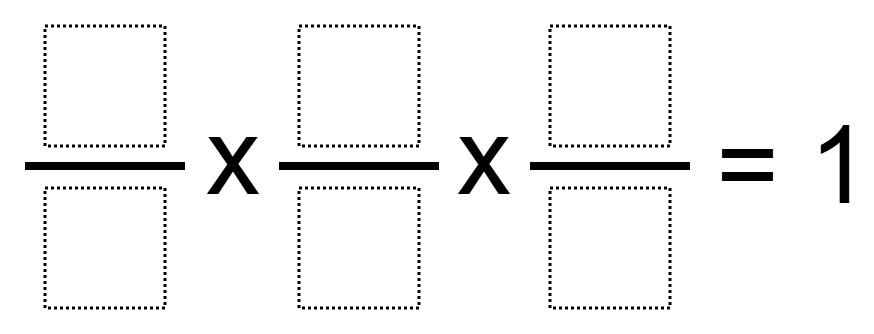# Fraction Multiplication Equal to 1

Directions: Use the digits 1 to 9, at most one time each, to fill in the boxes so that the three fractions have a product as close to 1 as possible.### Hint

What must be true about the numerator and denominator for the final result to be equal to 1?

There are many answers but all of them will either be 36/36 or 72/72 when multiplied out. For example, 6/9 x 4/8 x 3/1 = 72/72 = 1

Source: Patrick Vennebush

## Add Fractions with Decimal Sums

Directions: Using the digits 1 to 9 at most one time each, place a digit …

1.••